## 【数据结构】栈和队列（笔记总结）

article2023/10/1 11:14:11/👦个人主页：@Weraphael
✍🏻作者简介：目前学习C++和算法
✈️专栏：数据结构
🐋 希望大家多多支持，咱一起进步！😁

【本章内容】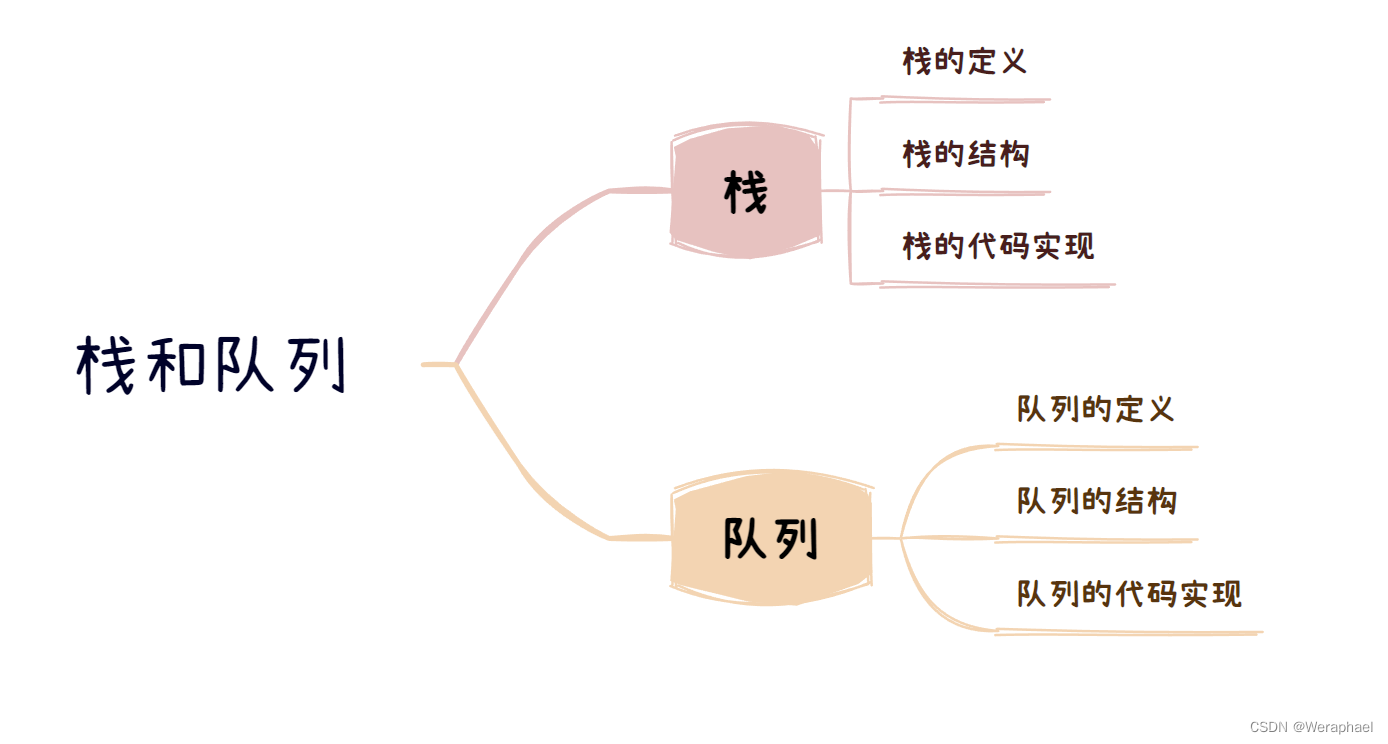### 目录

• 一、栈
• 1.1 概念
• 1.2 栈的结构
• 1.3 准备工作
• 1.4 常见接口
• 1.5 代码实现之栈的初始化
• 1.6 代码实现之栈的销毁
• 1.7 代码实现之栈的尾插
• 1.8 代码实现之栈的尾删
• 1.9 代码实现之栈的大小
• 1.9 代码实现之判断栈是否为空
• 1.10 代码实现之返回栈顶元素
• 1.11 test.c
• 二、队列
• 2.1 概念
• 2.2 队列的结构
• 2.3 准备工作
• 1.4 常见接口
• 1.5 队列之头尾指针初始化
• 1.5 队列之内存空间的销毁
• 1.6 队列之尾插
• 1.7 队列之头删
• 1.8 队列之求队列大小
• 1.9 队列之判断队列是否为空
• 1.10 队列之求队头元素
• 1.11 队列之求队尾元素
• 1.12 test.c部分

# 一、栈

### 1.1 概念

• 栈是一种特殊的线性表只允许在固定的一端进行插入和删除元素的操作。其中进行数据插入和删除操作的一端称为栈顶，另一端称为栈底。栈中的元素遵守后进先出LIFO(Last In First Out)的原则。
• 压栈：栈的插入操作叫做进栈/压栈/入栈，入数据在栈顶
• 出栈：栈的删除操作叫做出栈，出数据也是在栈顶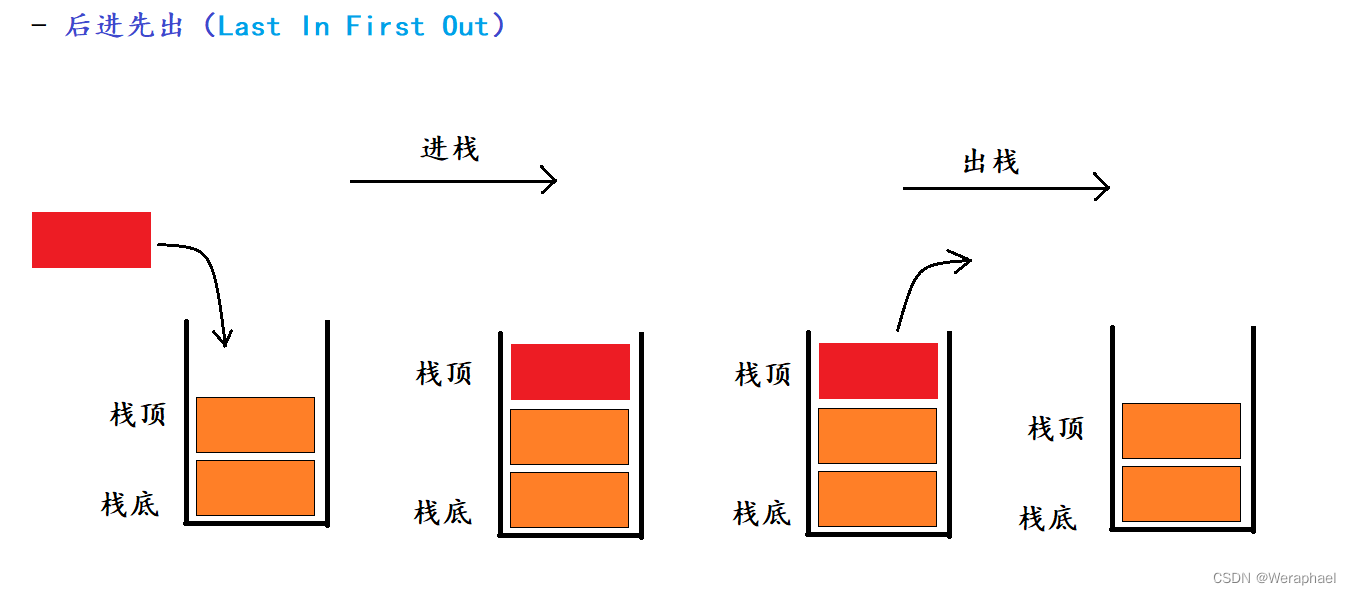### 1.2 栈的结构

【结构】

``````typedef struct Stack
{
int* a;  	//指向动态开辟的数组
int top; 	//表示栈顶
int capacity;//容量空间的大小
}Stack;
``````

### 1.3 准备工作

test.c - 测试代码逻辑 （源文件）
stack.c - 动态的实现 （源文件）
stack.h - 存放函数的声明 （头文件）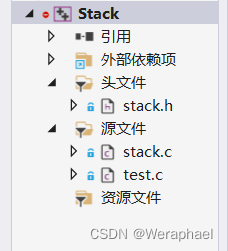### 1.4 常见接口

【stack.h】

``````typedef struct Stack
{
int* a;
int top;
int capacity;
}Stack;

//栈的初始化
void STInit(Stack* ps);

//栈的销毁
void STDestroy(Stack* ps);

//栈的尾插
void STPush(Stack* ps, int x);

//栈的尾删
void STPop(Stack* ps);

//栈的大小
int STSize(Stack* ps);

//栈是否为空
bool STEmpty(Stack* ps);

//栈顶元素
int STTop(Stack* ps);
``````

### 1.5 代码实现之栈的初始化

【stack.c】

``````//栈的初始化
void STInit(Stack* ps)
{
assert(ps);
ps->a = (int*)malloc(sizeof(int) * 4);
if (ps->a == NULL)
{
perror("ps->a :: malloc");
return;
}
ps->top = 0;
ps->capacity = 4;
}
``````
• 详细细节参考这篇博客： 点击跳转

### 1.6 代码实现之栈的销毁

【stack.c】

``````//栈的销毁
void STDestroy(Stack* ps)
{
assert(ps);

free(ps->a);
ps->a = NULL;

ps->capacity = 0;
ps->top = 0;
}
``````
• 详细细节参考这篇博客： 点击跳转

### 1.7 代码实现之栈的尾插

【stack.c】

``````//栈的尾插
void STPush(Stack* ps, int x)
{
assert(ps);
//判断是否需要扩容
if (ps->top == ps->capacity)
{
int* tmp = (int*)realloc(ps->a, sizeof(int) * ps->capacity * 2);
if (tmp == NULL)
{
perror("tmp :: realloc");
return;
}
ps->capacity *= 2;
ps->a = tmp;
tmp = NULL;
}
//尾插
ps->a[ps->top] = x;
ps->top++;
}
``````
• 详细细节参考这篇博客： 点击跳转

### 1.8 代码实现之栈的尾删

【stack.c】

``````//栈的尾删
void STPop(Stack* ps)
{
assert(ps);
//特判顺序表为空的情况
assert(!STEmpty(ps));

ps->top--;
}

``````
• 详细细节参考这篇博客： 点击跳转

### 1.9 代码实现之栈的大小

【stack.c】

``````//栈的大小
int STSize(Stack* ps)
{
assert(ps);

return ps->top;
}
``````

【学习笔记】

### 1.9 代码实现之判断栈是否为空

【stack.c】

``````//栈是否为空
bool STEmpty(Stack* ps)
{
assert(ps);
return ps->top == 0;
}
``````

### 1.10 代码实现之返回栈顶元素

【stack.c】

``````//栈顶元素
int STTop(Stack* ps)
{
assert(ps);
//当ps->top 为 0时，会导致越界
assert(ps->top != 0);//assert(!STEmpty(ps));

return ps->a[ps->top - 1];
}
``````

### 1.11 test.c

``````#include "stack.h"

int main()
{
Stack st;
STInit(&st);

STPush(&st, 1);
STPush(&st, 2);
STPush(&st, 3);
STPush(&st, 4);

//注意：栈不能直接打印，因为它在途中出数据
while (!STEmpty(&st))
{
printf("%d ", STTop(&st));
STPop(&st);
}

STDestroy(&st);

return 0;
}
``````

# 二、队列

### 2.1 概念

• 只允许在一端进行插入数据操作，在另一端进行删除数据操作的特殊线性表
• 队列具有先进先出FIFO(First In First Out)
• 入队列：进行插入操作的一端称为队尾
出队列：进行删除操作的一端称为队头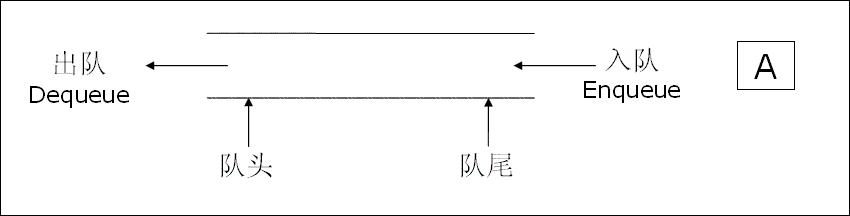### 2.2 队列的结构

【结构】

``````typedef struct QNode
{
struct QNode* next;
int data;
}QNode;

typedef struct Queue
{
QNode* tail;
int size;
}Queue;
``````

### 2.3 准备工作

test.c - 测试代码逻辑 （源文件）
Queue.c - 动态的实现 （源文件）
Queue.h - 存放函数的声明 （头文件）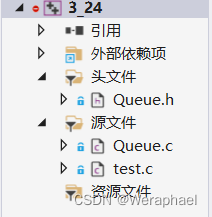### 1.4 常见接口

【Queue.h】

``````typedef struct QNode
{
struct QNode* next;
int data;
}QNode;

typedef struct Queue
{
QNode* tail;
int size; // 队列大小
}Queue;

//头指针和尾指针的初始化
void QueueInit(Queue* pq);

//开辟内存空间的销毁
void QueueDestroy(Queue* pq);

//队列的尾插
void QueuePush(Queue* pq, int x);

//队列的头删
void QueuePop(Queue* pq);

//队列的大小
int QueueSize(Queue* pq);

//判断队列是否为空
bool QueueEmpty(Queue* pq);

//队头数据
int QueueFront(Queue* pq);

//队尾数据
int QueueBack(Queue* pq);
``````

### 1.5 队列之头尾指针初始化

``````//头指针和尾指针的初始化
void QueueInit(Queue* pq)
{
assert(pq);
pq->size = 0;
}
``````

【笔记总结】

1. 断言问题。`pq`是一个结构体指针，指向一个首尾指针的结构体，`pq`指向`NULL`，这个队列就没法玩了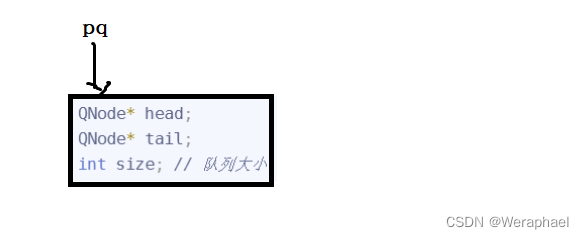### 1.5 队列之内存空间的销毁

``````//开辟内存空间的销毁
void QueueDestroy(Queue* pq)
{
assert(pq);
while (cur)
{
//在删除当前节点前记录下一个节点
QNode* next = cur->next;
free(cur);
cur = next;
}
pq->size = 0;
}
``````

### 1.6 队列之尾插

``````//队列的尾插
void QueuePush(Queue* pq, int x)
{
assert(pq);
//尾插的第一步，先向内存申请空间
QNode* newnode = (QNode*)malloc(sizeof(QNode));
if (newnode == NULL)
{
perror("newnode :: malloc");
return;
}
//再对newnode初始化
newnode->data = x;
newnode->next = NULL;

//接下来开始尾插
//第一个问题：当前的链表可能为空
{
//在链表为空的情况下tail，也一定为空（特判）
//若tail不为空就是传错了
assert(pq->tail == NULL);
//直接赋值即可
}
//否则就是正常的尾插
else
{
//tail newnode
pq->tail->next = newnode;
pq->tail = newnode; //更新tail
}
//尾插后size个数+1
pq->size++;
}
``````

### 1.7 队列之头删

``````//队列的头删
void QueuePop(Queue* pq)
{
assert(pq);
//空链表是不能头删的

//头删的特殊情况：链表中只有一个节点
{
}
//接下来就是正常的头删
else
{
//记录头节点的下一个节点
}
//删掉一个元素，队列的个数就要减1
pq->size--;
}
``````

### 1.8 队列之求队列大小

``````//队列的大小
int QueueSize(Queue* pq)
{
assert(pq);
return pq->size;
}
``````

### 1.9 队列之判断队列是否为空

``````//判断队列是否为空
bool QueueEmpty(Queue* pq)
{
assert(pq);
return pq->size == 0;
}
``````

### 1.10 队列之求队头元素

``````//队头数据
int QueueFront(Queue* pq)
{
assert(pq);
assert(!QueueEmpty(pq));
}
``````

### 1.11 队列之求队尾元素

``````//队尾数据
int QueueBack(Queue* pq)
{
assert(pq);
assert(!QueueEmpty(pq));
return pq->tail->data;
}

``````

### 1.12 test.c部分

``````#include "Queue.h"

int main()
{
Queue node;
QueueInit(&node);

//入队列（尾插）
QueuePush(&node, 1);
QueuePush(&node, 2);
QueuePush(&node, 3);
QueuePush(&node, 4);

//注意：队列不能直接打印，因为它在途中出数据
while (!QueueEmpty(&node))
{
printf("%d ", QueueFront(&node));
QueuePop(&node);
}
printf("\n");

QueueDestroy(&node);
return 0;
}
``````

### 【Matlab算法】粒子群算法求解二维线性优化问题（附MATLAB代码）

MATLAB求解二维线性优化问题前言正文函数实现可视化结果前言 二维线性优化问题指的是在二维空间中&#xff0c;对于一个由线性函数构成的目标函数&#xff0c;通过限制自变量的范围或满足特定的约束条件&#xff0c;寻找一个最优解&#xff08;最小值或最大值&#xff09;。这…

### Windows上提示 api-ms-win-core-path-l1-1-0.dll 丢失怎么办？

Windows上提示 api-ms-win-core-path-l1-1-0.dll 丢失怎么办&#xff1f;最近有用户在开启电脑的photoshop软件使用的时候&#xff0c;出现另外无法启动软件的情况&#xff0c;因为系统中缺失了对应的dll文件。那么这个情况怎么去进行问题的解决呢&#xff1f;来看看以下的解决…

### JUC并发编程第一章之进程/并发/异步的概念[理解基本概念]

1. 进程和线程的概念 进程: 系统正在运行的一个应用程序;程序一旦运行就是一个进程;进程是资源分配的最小单位 线程: 是进程的实际运行单位;一个人进程可以并发控制多个线程,每条线程并行执行不同的任务 区别: 进程基本上相互独立的;而线程存在于进程内&#xff0c;是进程…

### 【Node.js】Express框架的基本使用

✍️ 作者简介: 前端新手学习中。 &#x1f482; 作者主页: 作者主页查看更多前端教学 &#x1f393; 专栏分享&#xff1a;css重难点教学 Node.js教学 从头开始学习 目录 初识Express Express简介 什么是Express 进一步理解 Express Express能做什么 Express的基本使用 …

### Midjourney 使用总结

1.关键词提问 中国古典女性&#xff0c;东方美女极致面容站在运河码头遥望丈夫&#xff0c;史诗奇幻场景风格&#xff0c;深绿浅棕&#xff0c;细节风帆&#xff0c;柔焦&#xff0c;人像隐喻&#xff0c;4K&#xff0c;电影级高品质照片&#xff0c;全景图&#xff0c; 焦距 …